## 形状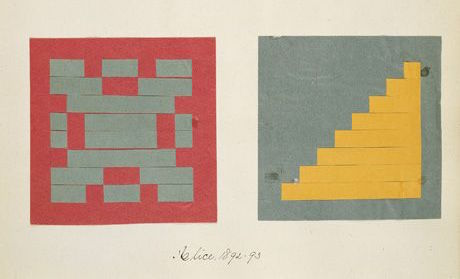### 长方形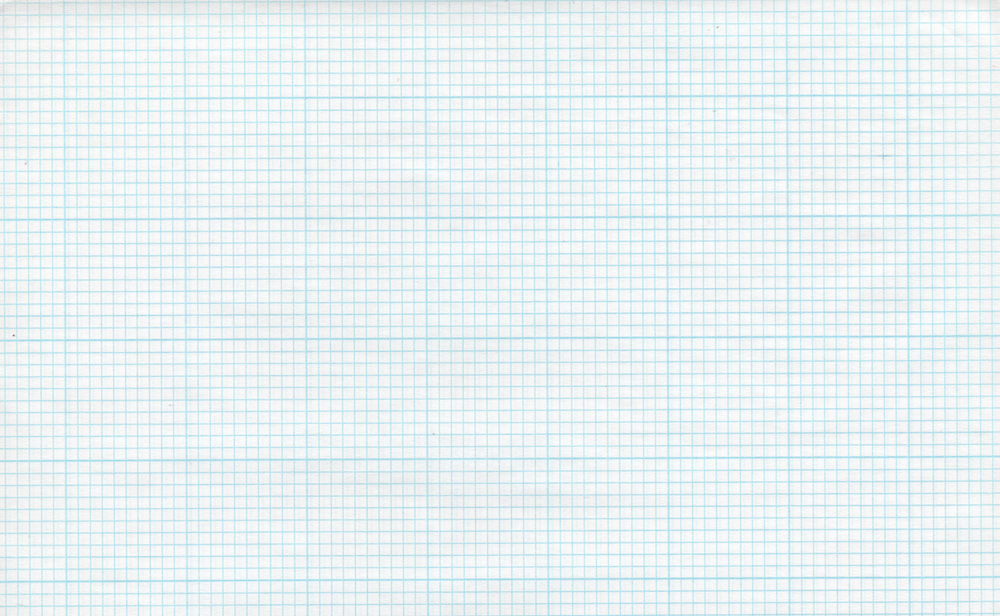``````    if ( (X GREATER THAN 1) AND (Y GREATER THAN 1) )
paint white
else
paint black``````

``````uniform vec2 u_resolution;

void main(){
vec2 st = gl_FragCoord.xy/u_resolution.xy;
vec3 color = vec3(0.0);

// Each result will return 1.0 (white) or 0.0 (black).
float left = step(0.1,st.x);   // Similar to ( X greater than 0.1 )
float bottom = step(0.1,st.y); // Similar to ( Y greater than 0.1 )

// The multiplication of left*bottom will be similar to the logical AND.
color = vec3( left * bottom );

gl_FragColor = vec4(color,1.0);
}``````

step（）函数会让每一个小于0.1的像素变成黑色（vec3（0.0））并将其余的变成白色（vec3（1.0））。`left``bottom` 效果相当于逻辑 AND —— 当 x y 都为 1.0 时乘积才能是 1.0。这样做的效果就是画了两条黑线，一个在画布的底边另一个在左边。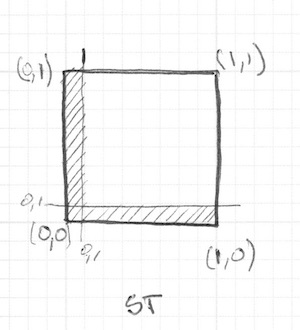``````    vec2 borders = step(vec2(0.1),st);
float pct = borders.x * borders.y;``````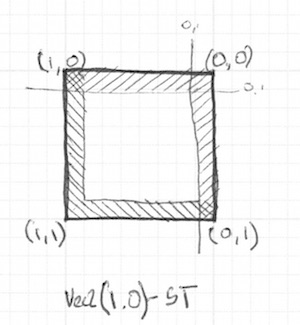``````    vec2 bl = step(vec2(0.1),st);       // bottom-left
vec2 tr = step(vec2(0.1),1.0-st);   // top-right
color = vec3(bl.x * bl.y * tr.x * tr.y);``````

• 改变长方形的比例和大小。

• 用 smoothstep() 函数代替 step() 函数，试试在相同的代码下会有什么不同。注意通过改变取值，你可以不仅可以得到模糊边界也可以由漂亮的顺滑边界。

• 应用 floor() 做个另外的案例。

• 挑个你最喜欢的做成函数，这样未来你可以调用它。并且让它灵活高效。

• 写一个只画长方形四边的函数。

• 想一下如何在一个画板上移动并放置不同的长方形？如果你做出来了，试着像Piet Mondrian一样创作以长方形和色彩的图画。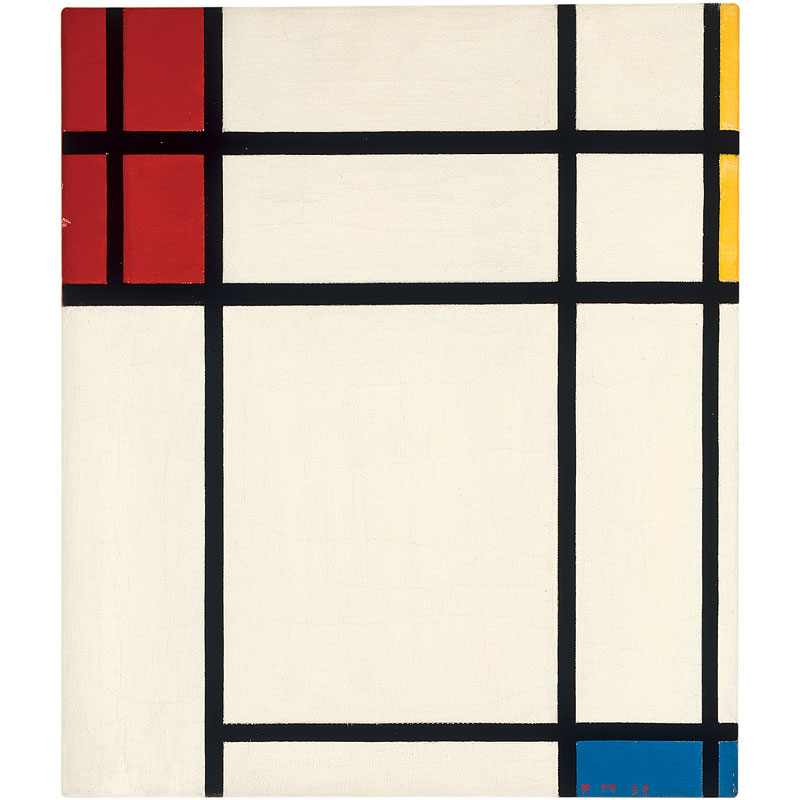### 圆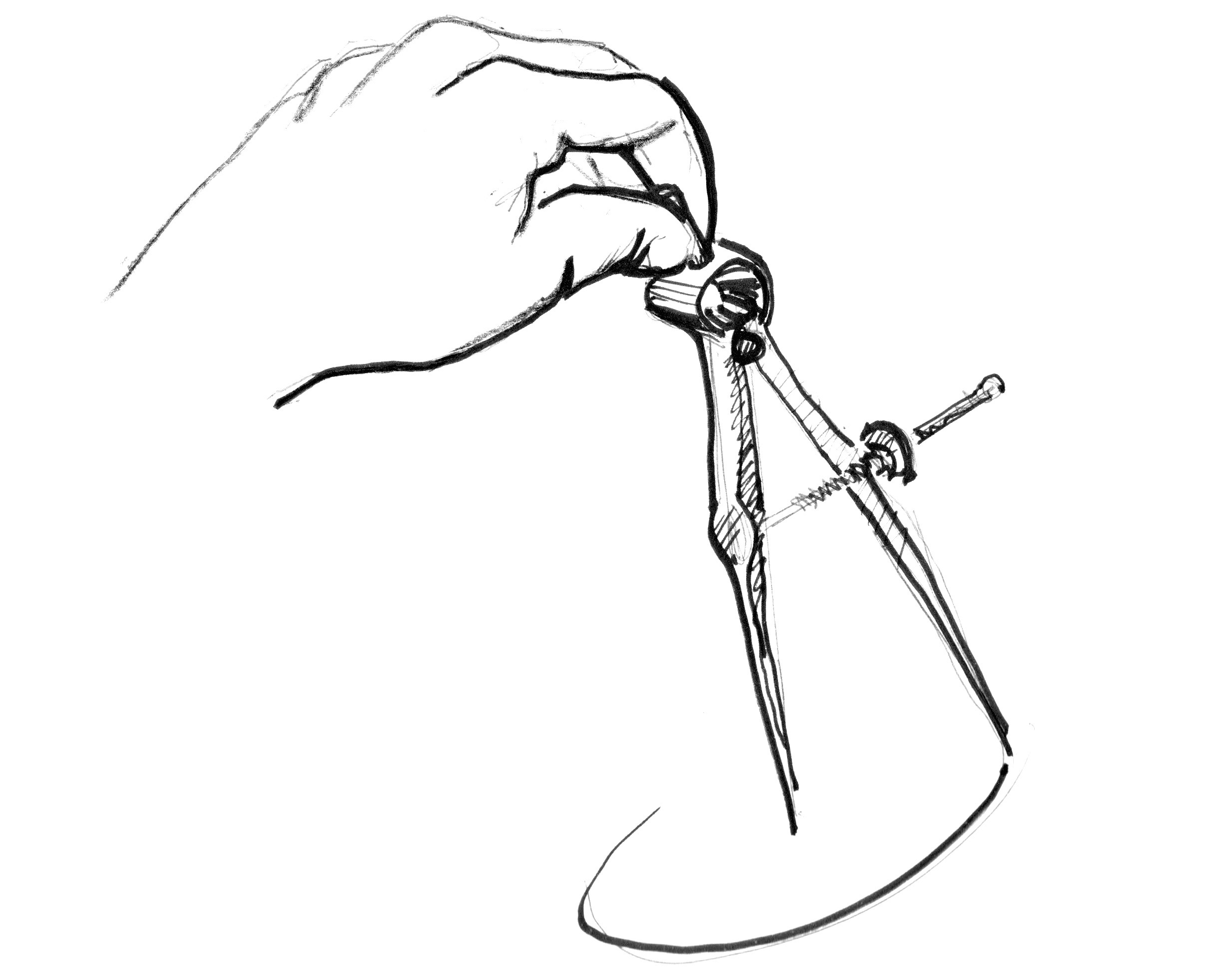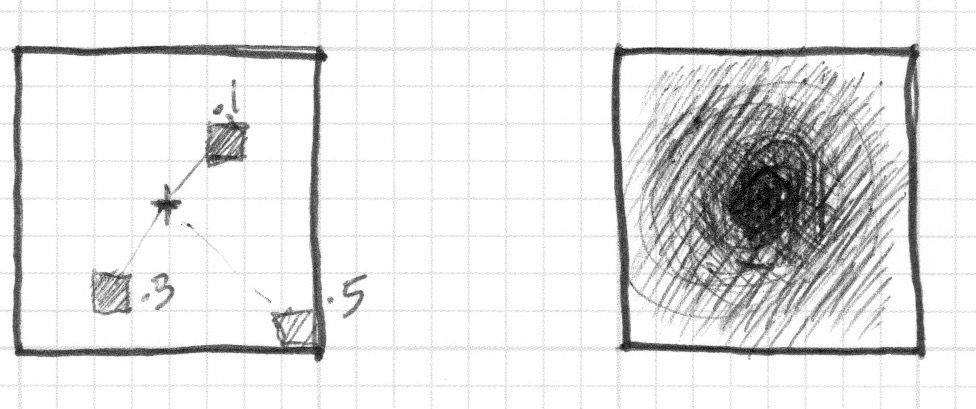There are several ways to calculate that distance. The easiest one uses the `distance()` function, which internally computes the `length()` of the difference between two points (in our case the pixel coordinate and the center of the canvas). The `length()` function is nothing but a shortcut of the hypotenuse equation that uses square root (`sqrt()`) internally.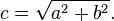• 注释和取消某行的注释来试试看用不同方式得到相同的结果。

• 你能从中推断出什么？

• 我们怎么用这个方法来画圆？

• 试试有没有其他方法来实现这样画布内圆形渐变的效果。

### 距离场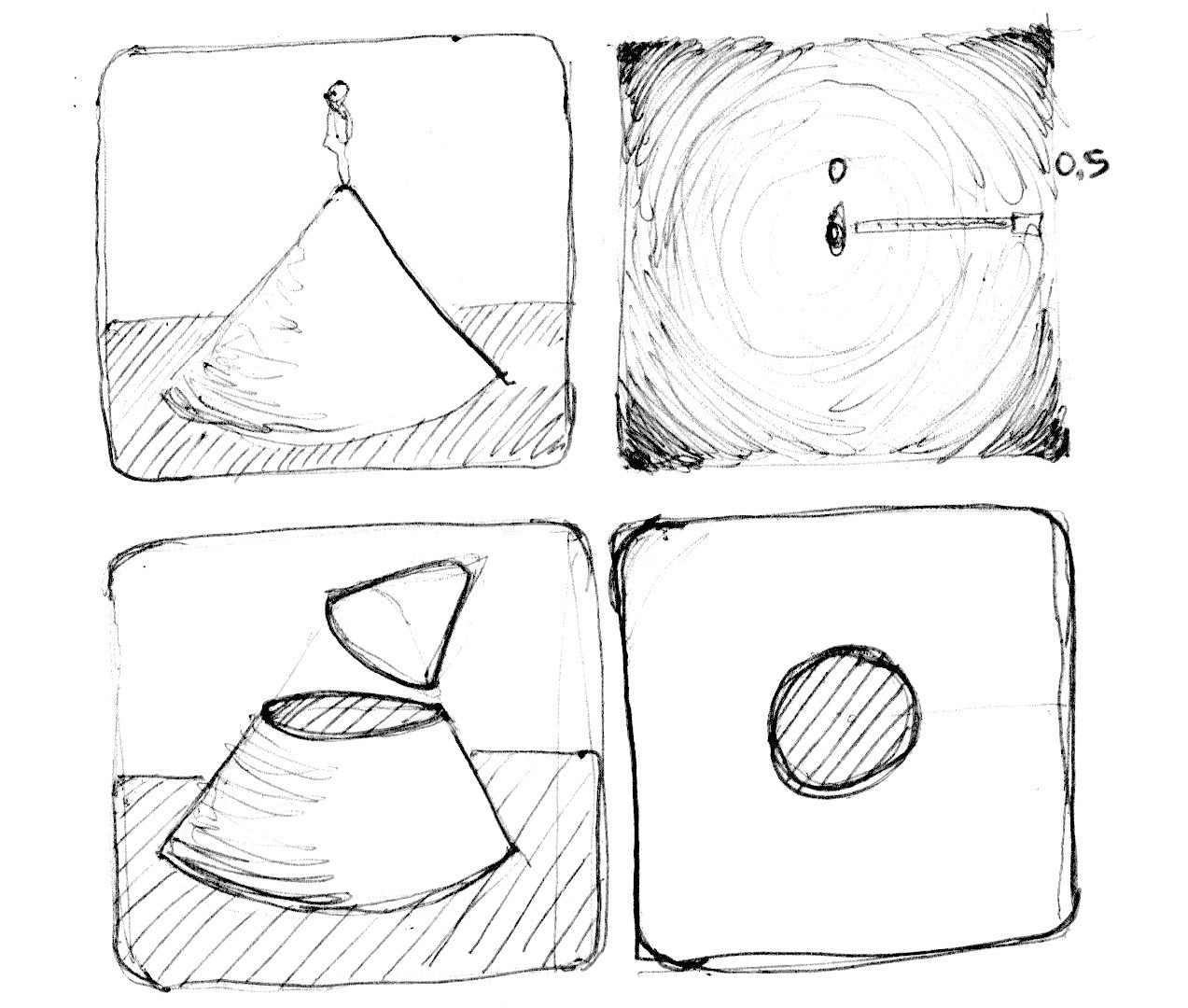• `step()`函数把所有大于0.5的像素点变成白色，并把小于的变成黑色（0.0）。

• 反转前景色和背景色。

• 使用`smoothstep()`函数，通过修改不同的值来试着做出一个边界顺滑的圆。

• 一旦遇到令你满意的应用，把他写成一个函数，这样将来就可以调用了。

• 给这个圆来些缤纷的颜色吧！

• 再加点动画？一闪一闪亮晶晶？或者是砰砰跳动的心脏？（或许你可以从上一章汲取一些灵感）

• 让它动起来？能不能移动它并且在同一个屏幕上放置多个圆？

• 如果你结合函数来混合不同的距离场，会发生什么呢？
``````pct = distance(st,vec2(0.4)) + distance(st,vec2(0.6));
pct = distance(st,vec2(0.4)) * distance(st,vec2(0.6));
pct = min(distance(st,vec2(0.4)),distance(st,vec2(0.6)));
pct = max(distance(st,vec2(0.4)),distance(st,vec2(0.6)));
pct = pow(distance(st,vec2(0.4)),distance(st,vec2(0.6)));``````
• 用这种技巧制作三个元素，如果它们是运动的，那就再好不过啦！

### 距离场的特点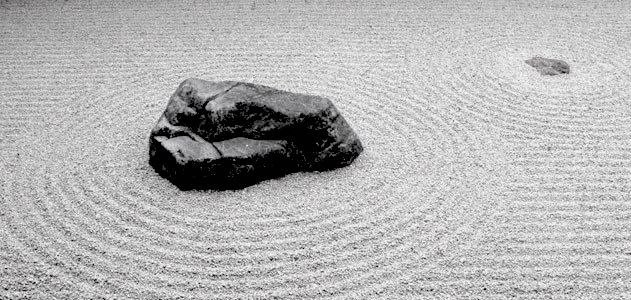### 极坐标下的图形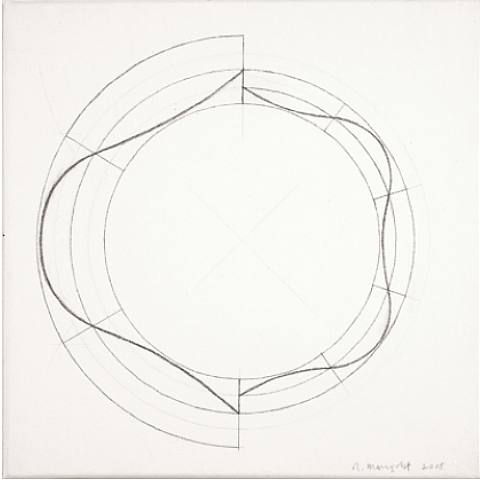``````    vec2 pos = vec2(0.5)-st;
float r = length(pos)*2.0;
float a = atan(pos.y,pos.x);``````

• 让这些图形动起来。
• 结合不同的造型函数来 雕刻 图形，制作诸如花，雪花和齿轮。
• 用我们在 造型函数 章节的 `plot()` 函数画等高线。

### 整合的魅力

• 用这个例子，改造一个输入位置，指定图形（形状）的顶点数来返回一个距离场（的值）。

• 结合使用 `min()``max()` 函数混合距离场。

• 用距离场画个自己感兴趣的logo。